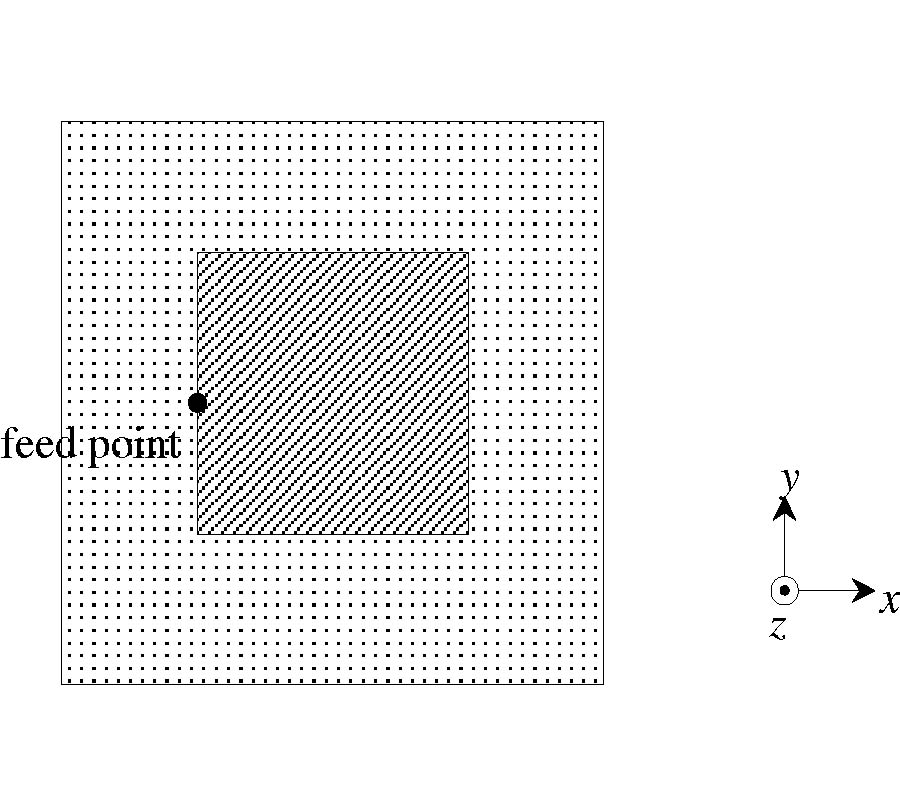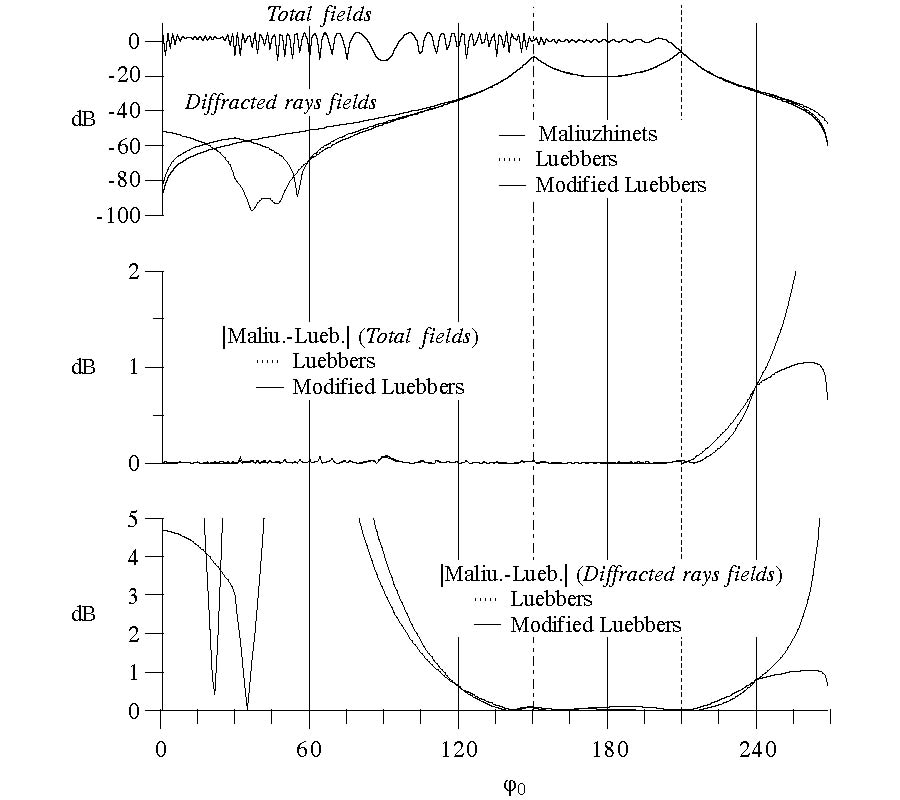Vol. 33
Latest Volume
All Volumes
All Issues
0000-00-00
PIER
Vol. 33, 299-331, 2001
Modeling of Rectangular Waveguide Junctions Containing Cylindrical Posts
Kawthar Zaki
Accurate determination of the generalized scattering matrix of the crossed waveguides junction, T-junction and right-angle bend containing a cylindrical post is presented. The generalized scattering matrix of the four port structure is obtained from those of the two ports right angle bend with different combinations of perfect electric and/or magnetic walls. The generalized scattering matrix of the right angle bend (quarter of the structure) is obtained by the mode matching method where the electromagnetic fields in rectangular waveguides are matched to those in a junction section formed by asectoral region.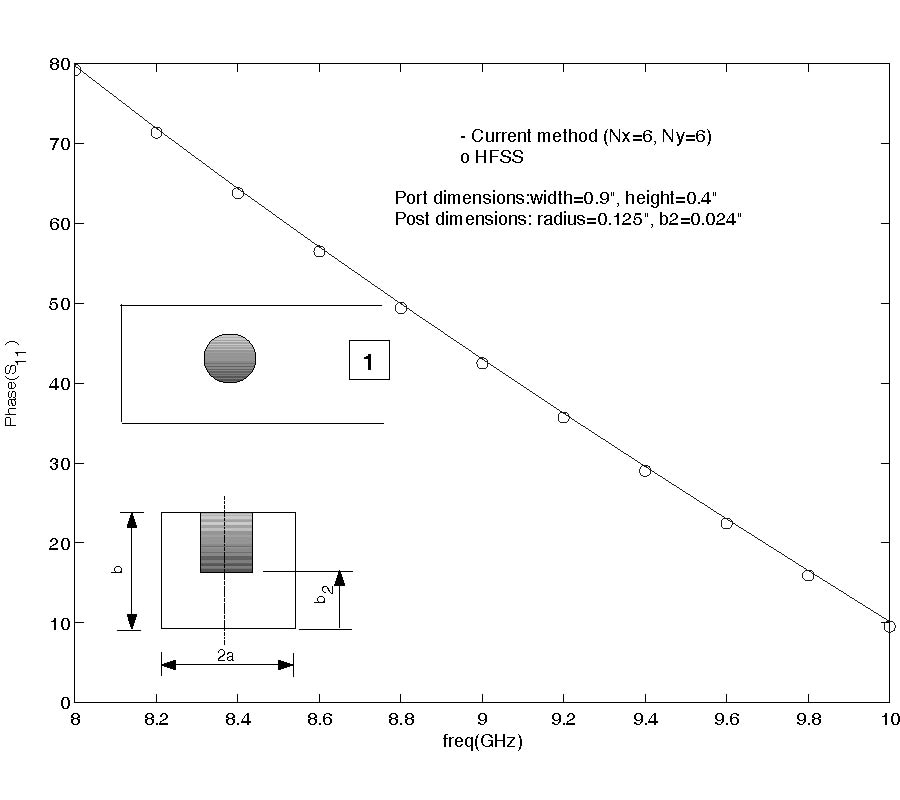PIER
Vol. 33, 261-298, 2001
Plane Wave Scattering by an Achiral Multilayered Sphere in an Infinitely Extended Chiral Host Medium
L.-W. Li M.-S. Leong T. S. Yeo J. A. Kong
An analytic solution to the problem of plane wave scattering by an achiral multilayered sphere in a host chiral medium is obtained in this paper. By applying the radiation-to-scattering transform, the scattering problem can be considered as the specific radiation problems where the radiated source equivalent to the electromagnetic plane wave is located at infinity. The volumetric currents which generate right circular polarization (RCP) and left circular polarization (LCP) plane waves,resp ectively, are found. An integral equation consisting the volumetric current distributions and the dyadic Green's functions is formulated to obtain both the equivalent incident wave fields and the scattered fields. Two-layered lossless and lossy dielectric spheres and a conducting sphere with a dielectric coated layer buried in an infinitely extended host chiral medium are considered and the expressions for the scattered fields in far-zone are found in explicit analytic form. The characteristics of scattered fields are illustrated and discussed in terms of the circular polarization degree and linear polarization degree against different chiral admittances and sizes.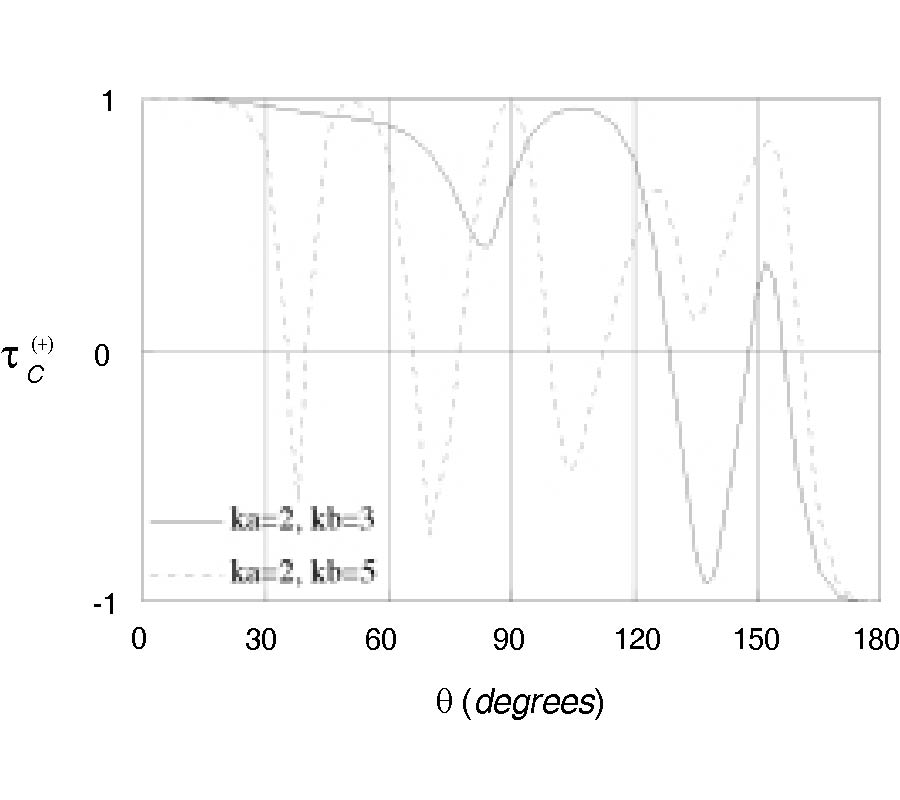PIER
Vol. 33, 237-259, 2001
The Influence of the Finite Size of the Illuminated Area on Electromagnetic Scattering from Surfaces with and Without Slicks
Alla I. Timchenko Klaus Schunemann
In this paper, the problem of scattering from sea surface with and without oil slicks is investigated taking the finite size of the illuminated area into account. A model of an inhomogeneous random rough surface with finite size of the scattering area is considered. To apply the results for a broad range of the random surface spectrum, an approach is developed which extends the range of validity beyond that of small perturbation theory. The general expression obtained for the scattering cross section takes into account a modulation of the rough surface by long surface waves. Analytical and numerical studies of the scattering cross section are provided to investigate the role of different mechanisms of scattering from various parts of the surface spectrum, and of diffraction caused by the finite size of the area. It is shown that the area size may affect the normalized scattering cross section in the case of the surface with a slick. Possibilities to explain the features of the suppression of the backscattering by oil slicks 238 Timchenko, Serebryannikov, and Schünemann are discussed. Furthermore, a way to distinguish between different scattering mechanisms is suggested.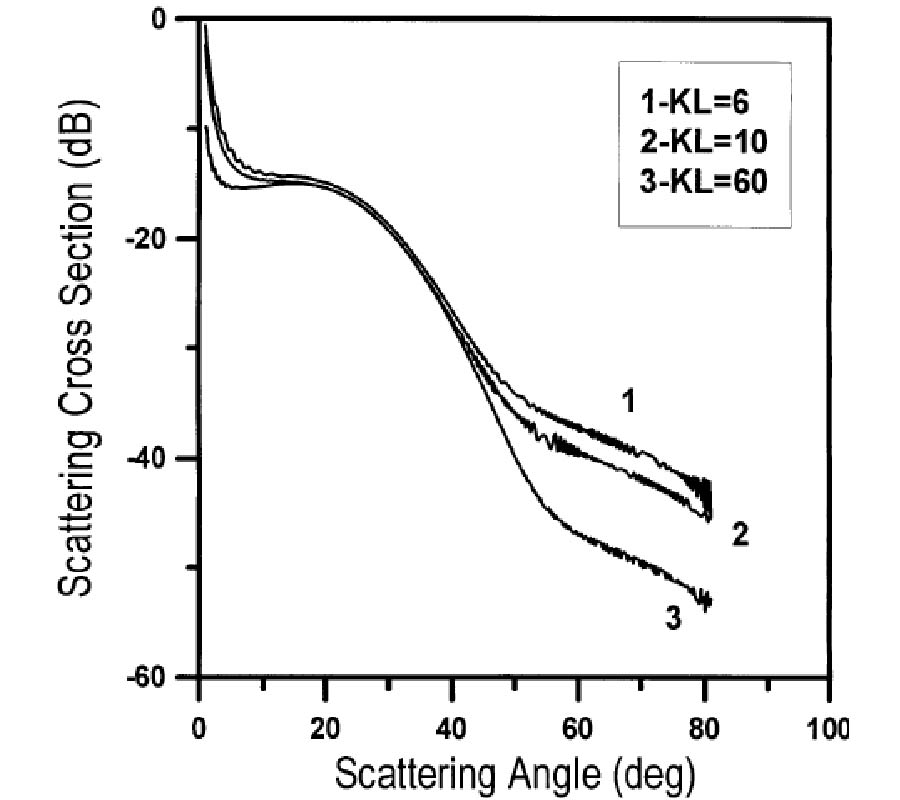PIER
Vol. 33, 219-235, 2001
Analytical Asymptotic Extraction Technique for the Analysis of Bend Discontinuity
Chan-Byoung Chae S.-O. Park Kyoung Whoan Suh
The purpose of this paper is to use the analytical asymptotic extraction technique to analyze the bend discontinuity. We show that the derived analytical techniques significantly reduce the computational time while improving the accuracy compared to the conventional method. Especially, the advantage of the proposed method can eliminate the truncation error for evaluating the asymptotic part of impedance matrix. The proposed method has applied for solving the bend discontinuity, and verified with measurement results.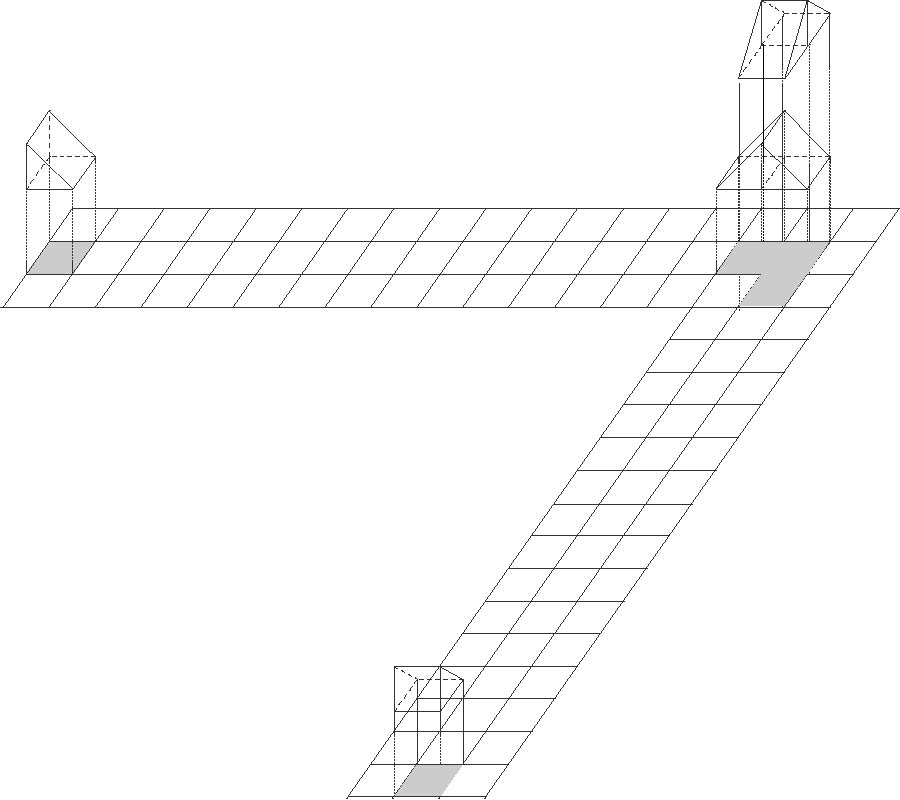PIER
Vol. 33, 199-218, 2001
Numerical Analysis of Scattered Power from a Layer of Random Medium Containing Many Particles of High Dielectric Constant -- Application to the Detection of a Water Content of Soil --
Tatsuro Matsuoka Mitsuo Tateiba
This paper shows the scattering cross sections of a random medium which is a simple model of moist soil by analyzing a dense medium radiative transfer equation (DMRT). The parameters in the DMRT, the extinction rate and the scattering coefficient, are calculated by a multiple scattering method called our method in this paper. Our method is valid for particles with high dielectric constant like water drops. Characteristics of the scattering cross section are made clear by changing the fractional volume of water and the incident angle, polarization of incident waves. We discuss the possibility of detection of a water content in this approach by using the characteristics of the scattering cross section.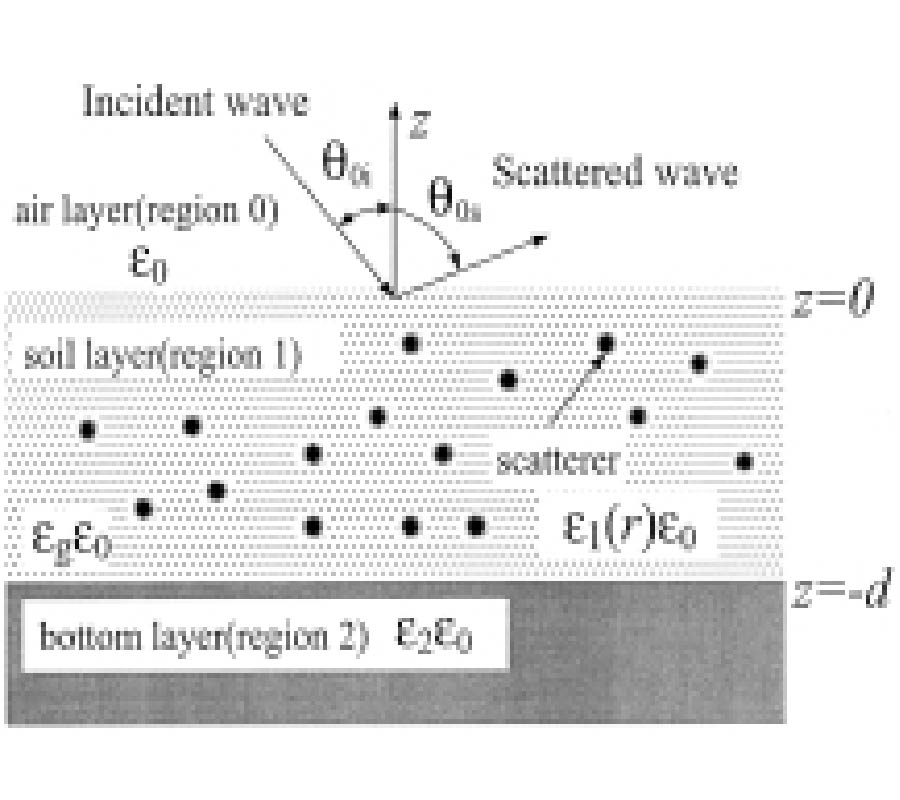PIER
Vol. 33, 183-197, 2001
Diffraction of Scalar Pulses at Plane Apertures: a Different Approach
Pierre Hillion
We generalize to scalar pulses with finite duration a previous work  in which a new approach to diffraction at plane apertures is developed for scalar harmonic waves. A particular attention is given to rectangular pulse modulated signals for which an exact solution to the diffraction problem is obtained. As an example, the diffraction of a truncated harmonic pulse is investigated and the numerical problems to be solved are discussed with an important simplification when one is only interested in the diffraction pattern far from the aperture. More works are needed for apertures with no simple geometrical form.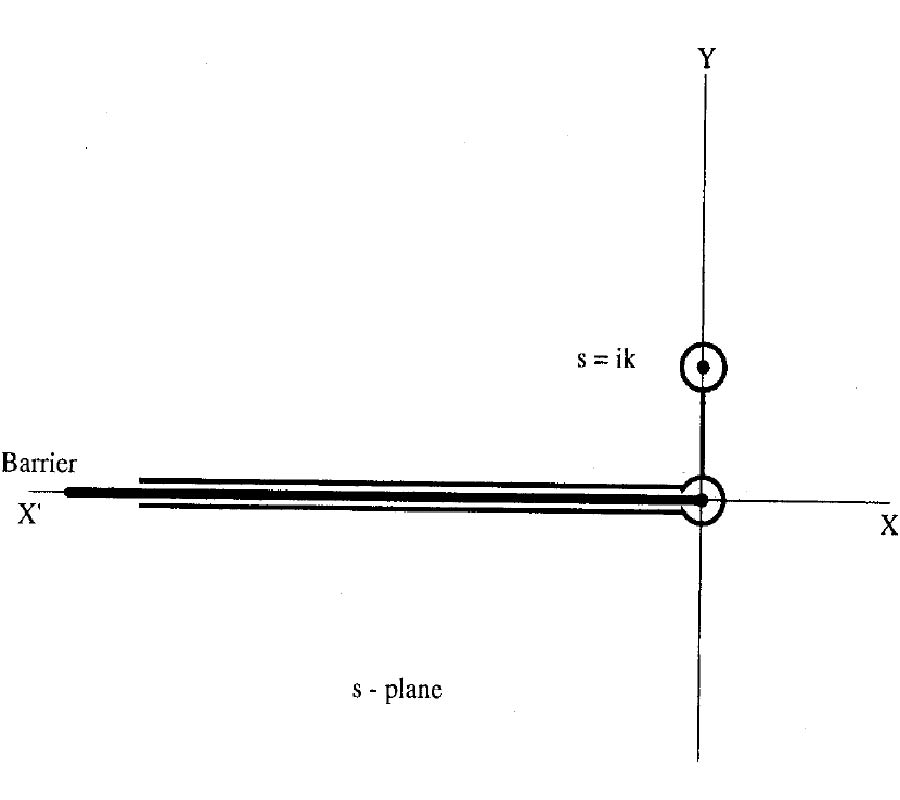PIER
Vol. 33, 167-182, 2001
Null Steering of Linear Antenna Arrays Using a Modified Tabu Search Algorithm
Kerim Guney Ali Akdagli
Auseful and flexible method based on the tabu search algorithm for the pattern synthesis of linear antenna arrays with the prescribed nulls is presented. Nulling of the pattern is achieved by controlling the amplitude-only and both the amplitude and phase of each array element. To show the versatility of the present method, some design specifications such as the sidelobe level, the null depth and the dynamic range ratio are considered by introducing a set of weighting factors in the cost function constructed for the tabu search algorithm. Several illustrative examples of Chebyshev pattern with the imposed single, multiple and broad nulls are given.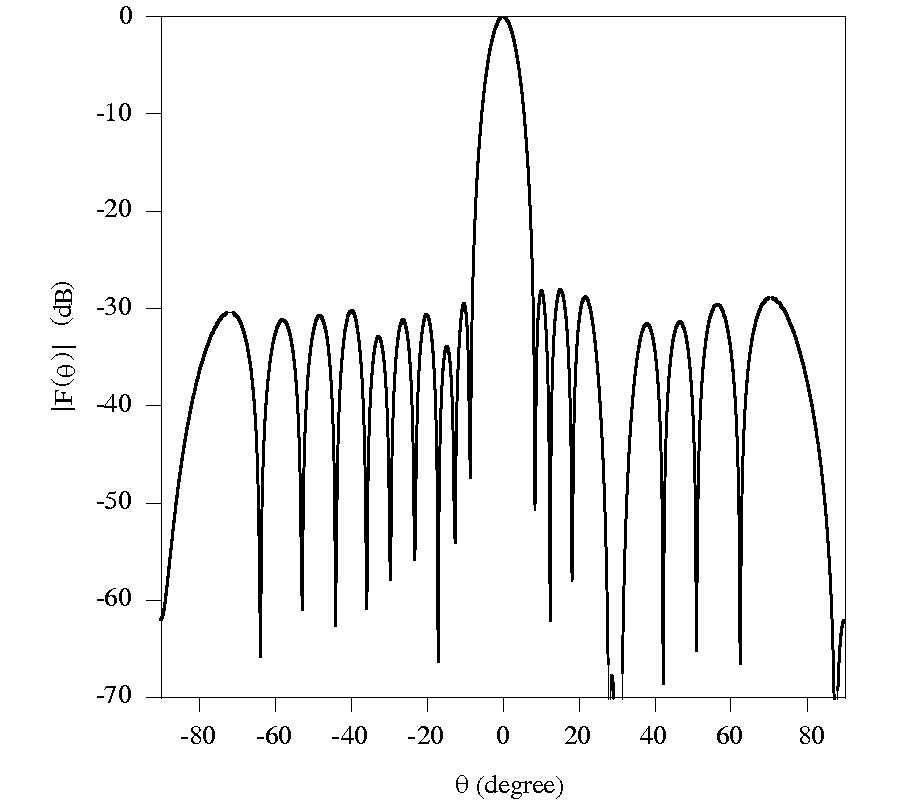PIER
Vol. 33, 141-165, 2001
Multilayered Media Green's Functions for Mpie with General Electric and Magnetic Sources by the Hertz Potential Approach
Pasi Yla-Oijala Matti Taskinen Jukka Sarvas
A complete set of three dimensional multilayered media Green's functions is presentedfor general electric andmagnetic sources. The Green's functions are derived in the mixed potential form, which is identical with the Michalski-Zheng C-formulation. The approach appliedin this paper is basedon the classical Hertz potential representation. A special emphasis is on the formulation of the dyadic Green's functions GHJ and GEM. In these functions the derivatives due to the curl operator are taken in the spectral domain. This avoids the needof the numerical differentiation. Furthermore, it is foundthat the Hertzian potentials satisfy several useful duality and reciprocity relations. By these relations the computational efficiency of the Hertz potential approach can be significantly improvedandthe number of requiredSommerfeldin tegrals can be essentially reduced. We show that all spectral domain Green's functions can be obtained from only two spectral domain Hertzian potentials, which correspond to the TE component of a vertical magnetic dipole and the TM component of a vertical electric dipole. The derived formulas are verified by numerical examples.PIER
Vol. 33, 119-139, 2001
Dynamics of Gaussian and Super-Gaussian Solitons in Birefringent Optical Fibers
The variational principle is employed to obtain the parameters dynamics of Gaussian and super-Gaussian chirped solitons which propagates through birefringent optical fibers that is governed by the dispersion-managed vector nonlinear Schrödinger's equation. The waveform deviates from that of a classical soliton. The periodically changing strong chirp of such a soliton reduces the effective nonlinearity that is necessary for balancing the local dispersion. This study is extended to obtain the adiabatic evolution of the parameters of such a soliton in presence of perturbation terms.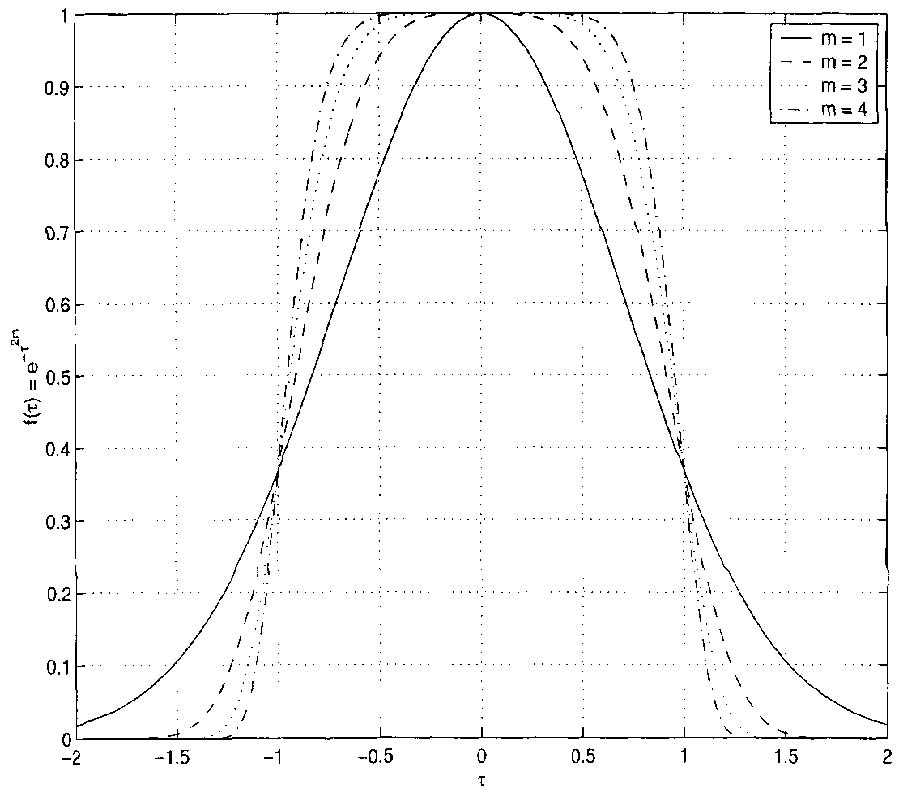PIER
Vol. 33, 97-118, 2001
Bandwidth Enhancement for Split Cylindrical Dielectric Resonator Antennas
Ahmed Kishk Allen Wilburn Glisson G. P. Junker
A numerical study of split cylindrical dielectric resonator antennas on a conducting ground plane excited by a coaxial probe is presented. The numerical solution is based on the method of moments for a body of revolution coupled to a wire. We consider in this study bandwidth enhancement for dielectric resonators excited in the HEM11 and HEM12 modes for the split dielectric cylinder. A wideband performance of about 35% has been achieved for the antenna and experimental measurements have verified this finding.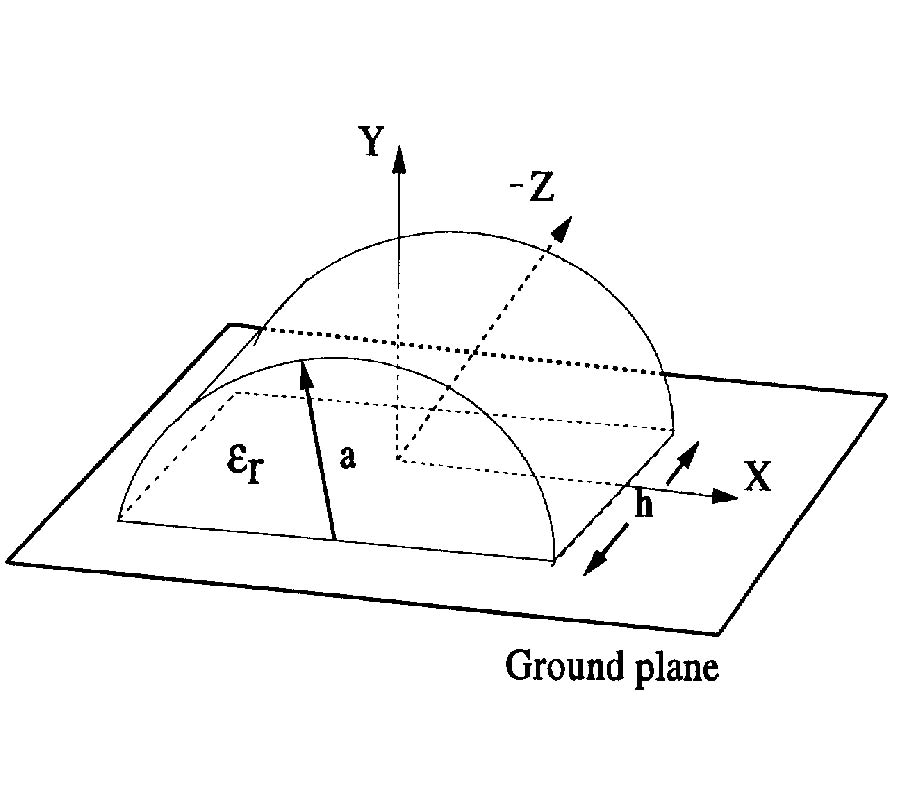PIER
Vol. 33, 69-96, 2001
On the Pulse Velocity in Absorbing and Nonlinear Media and Parallels with the Quantum Mechanics
I. Gurwich
A novel definition of pulse propagation velocity is introduced. It is shown that the present definition does not lead to confusing results such as complex velocity or velocity exceeding the light velocity in the vacuum. Also shown are the parallels of this definition to the classical and quantumm echanics conceptions. Using the present definition reveals certain analogies between electromagnetic pulse propagation in the classical physics and de Broglie wave-packets propagation in the quantumm echanics, thus adding support to its validity.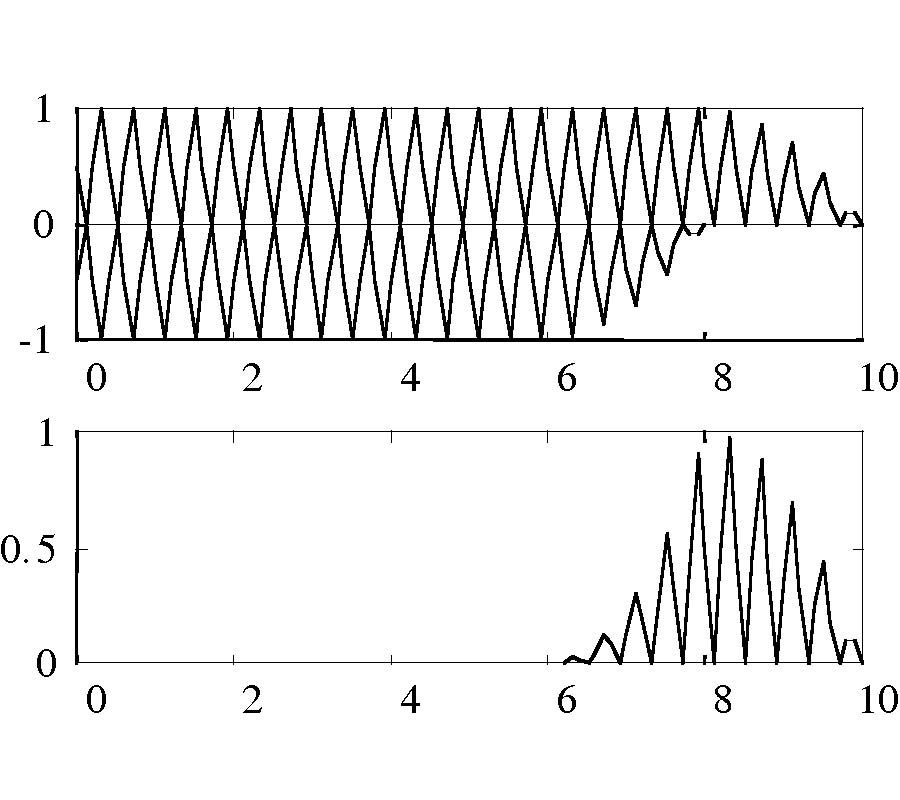PIER
Vol. 33, 45-68, 2001
Probabilistic Response of a Transmission Line in a Dissipative Medium Excited by an Oblique Plane Wave
P. Trakadas Panagiotis Papakanellos Christos N. Capsalis
In this paper a statistical model of the excitation of a conjugate-matched two-wire transmission line in a lossy half space by an electromagnetic (EM) wave is developed. The EM field, radiating in the air, is obliquely incident to the interface defined by the lossy medium and air. Three different orientations of the transmission line for horizontal and vertical polarization of the EM field are examined. The objective is to derive analytic formulas for the probability density function (pdf) and cumulative distribution function (cdf) of the induced near-end and far-end voltage magnitudes in each case, taking into consideration the statistical behavior of the amplitude of the incident electric field vector and the angle of incidence as well. Consequently, the mean values as well as the typical deviation values are presented and the contribution of each one of the parameters is discussed in detail. Finally, a chi-square goodness-of-fit test is applied in order to fit the distribution of the induced voltage with one of the known distributions.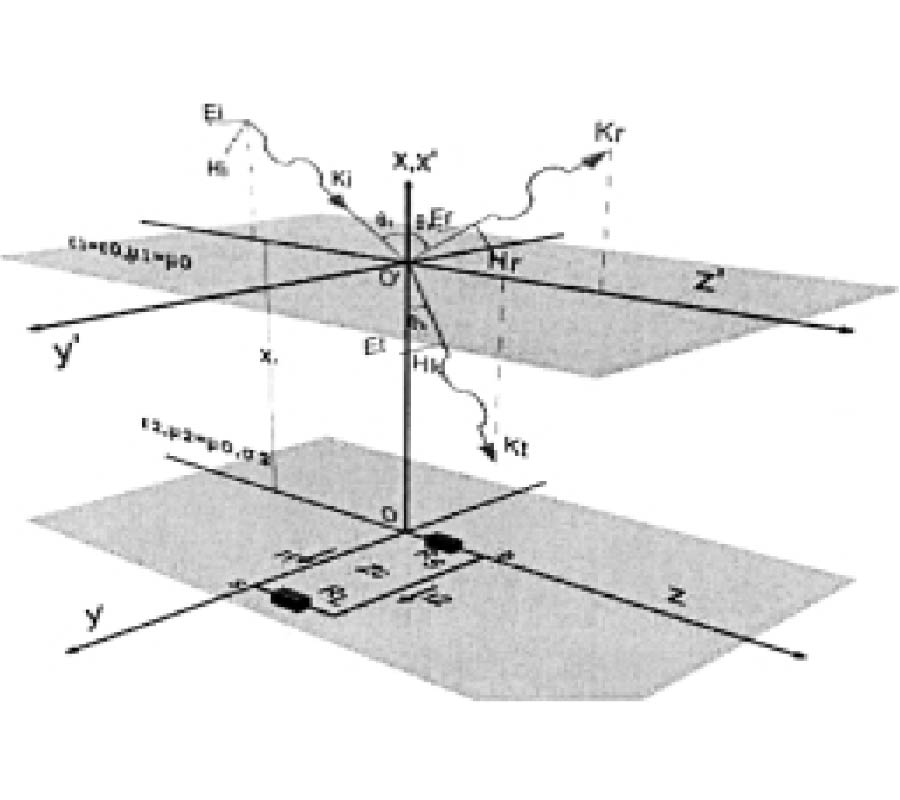PIER
Vol. 33, 29-43, 2001